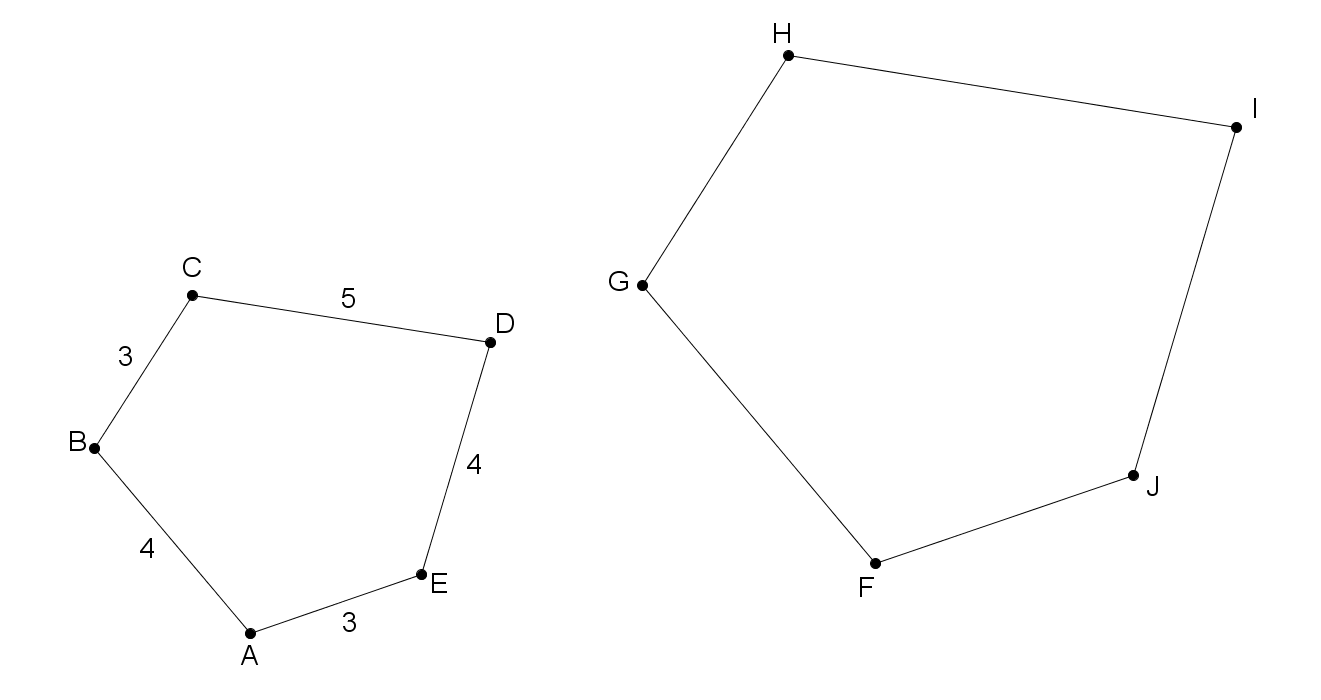# How Many Sides For Similarity?

Geometry Level 4In the figure above, $\angle A\cong\angle F$, $\angle B\cong\angle G$, $\angle C\cong\angle H$, $\angle D\cong\angle I$, and $\angle E\cong\angle J$.

You may set any side length in pentagon $FGHIJ$ to be whatever value you want. Your goal is to make the pentagons similar.

What is the minimum number of sides needed in order for both pentagons to always be similar?

×